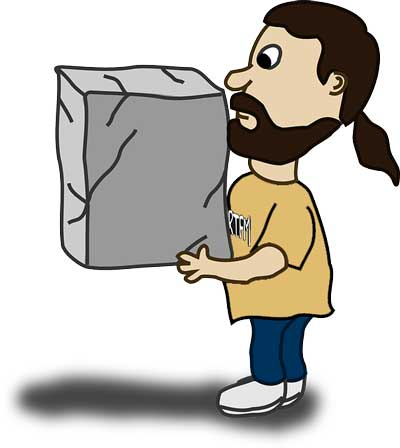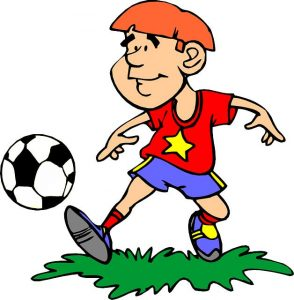In everyday life, we walk, drive cars, and lift objects. All these tasks need a force to be performed. So, it is necessary to understand this force scientifically. This force was described scientifically by Isaac Newton. He described the force and its relationships in his laws that are known today as the Newton’s Laws of Motion.

First Law of MotionThe first law states that an object in motion will continue to move with same speed in the same direction until another force acts on it. Furthermore, an object which is at rest (not moving) will not move until some external force acts on it.

You may be thinking, how this is possible. Because as you kick a ball, it will immediately start to slow down and will come to rest at a distance. This happens because of air friction. As you kick a ball, frictional forces immediately start to oppose the motion of the ball until it comes to rest.

Second Law of MotionThe Second Law states that force is equal to mass times the acceleration. Let’s say it in other words, the more force is applied to an object the more acceleration will be produced in it. As a result, the object will speed up faster. For example, if you hit a ball harder, it will go faster and farther.

The Second law of motion can also be defined in the mathematical form. It is defined as, F = mass * acceleration.

F = m*a; m is the mass and “a” is the acceleration

Third Law of Motion (Every action has reaction)

You may be familiar with the statement of this law which states that “every action has equal and opposite reaction”. In other words, each applied force also has the opposing force, which has the same magnitude of applied force but in opposite direction. For example, when you kick a ball with 10 N force, your feet will also experience the same 10 N force from the ball.

Facts

• First law of motion also tells us that, mass has a resistance to change in motion. This resistance is called inertia.
• Sir Isaac Newton is also the founder of Calculus; a branch of mathematics which deals with the rate of change.
• An object in motion experiences friction from the ground and air. Friction also helps our cars to slow down, when the brakes are pressed.